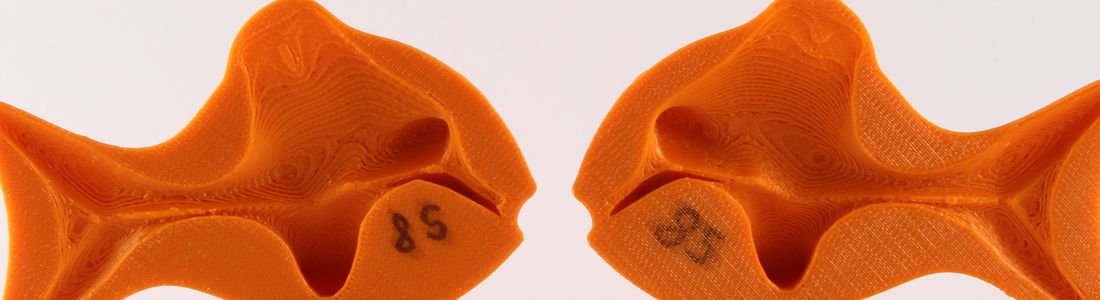# GCD Seminar

Map Unavailable

Date/Time
Date(s) - 14/03/2018
2:15 pm - 3:15 pm

Categories

Talk by Franz Pedit: “Gradient flows of geometric variational problems: the elastic curve flow

Abstract:
When considering a variational problem, one is interested in its minimizers, and more generally its critical points given by the Euler-Lagrange equation. The critical points are typically special geometries — Einstein metrics, Yang-Mills fields, harmonic maps, elastic membranes etc. — and one would like to flow a generic initial geometry to a terminal special geometry. This is usually accomplished by considering the gradient flow of the functional at hand. For instance, Riemann used heat flow to minimize the Dirichlet energy, thereby showing the existence of harmonic functions; the Ricci flow limits to Einstein metrics, which was eventually used to solve the Poincare conjecture. The definition of the gradient requires a choice of (Riemannian) metric on the domain of the functional, which to a large extent determines the analytical properties of the gradient flow.

In this lecture, we will discuss these issues in the simplest example (understandable to students at any level) of closed planar curves under the influence of the elastic energy, the average squared curvature of the curve. The critical points are the so-called elastica found by Leonard Euler in a beautiful paper of 1744. The usual gradient flow with respect to the variational \$L^2\$ metric is a 4th order non-linear parabolic PDE requiring hard analysis. We show that by using a geometrically defined metric, the gradient flow becomes a proper ODE on a Hilbert manifold, whose longtime existence and convergence to elastica requires nothing more than elementary ODE theory. We will intersperse the talk with numerical experiments carried out via this approach.

GCD Seminar